# Balancing Equations Balancing Writing and Naming Equations Balancing

• Slides: 51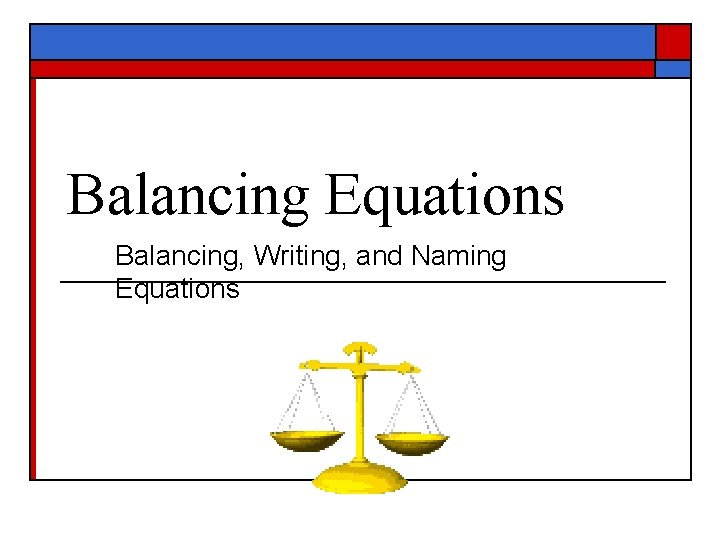Balancing Equations Balancing, Writing, and Naming EquationsBalancing EquationsBalancing Equations o Law of Conservation of Matter: n n n In a chemical reaction, matter can be neither created nor destroyed. In a chemical reaction, the amount of reactants equal the amount of products.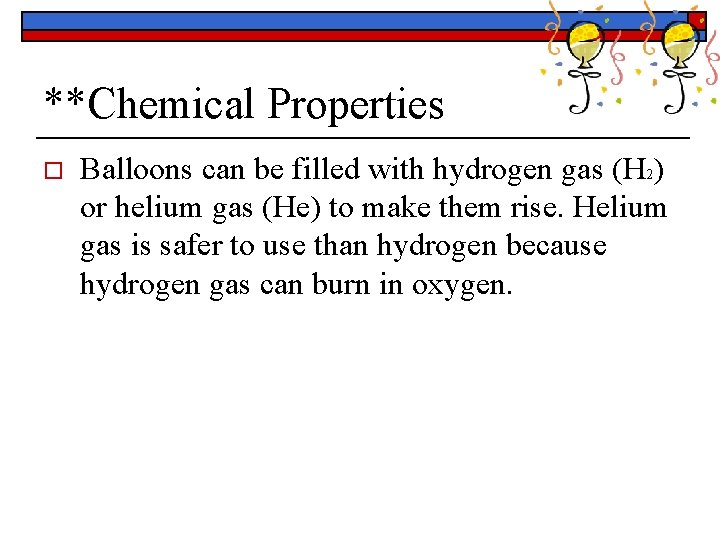**Chemical Properties o Balloons can be filled with hydrogen gas (H 2) or helium gas (He) to make them rise. Helium gas is safer to use than hydrogen because hydrogen gas can burn in oxygen.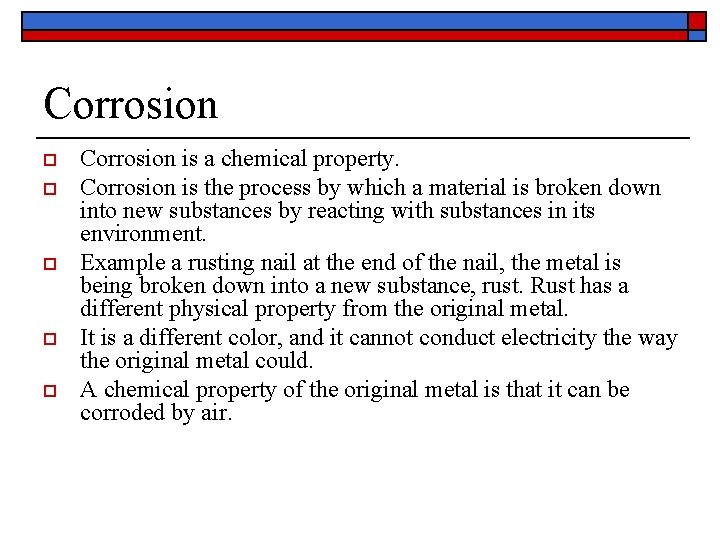Corrosion o o o Corrosion is a chemical property. Corrosion is the process by which a material is broken down into new substances by reacting with substances in its environment. Example a rusting nail at the end of the nail, the metal is being broken down into a new substance, rust. Rust has a different physical property from the original metal. It is a different color, and it cannot conduct electricity the way the original metal could. A chemical property of the original metal is that it can be corroded by air.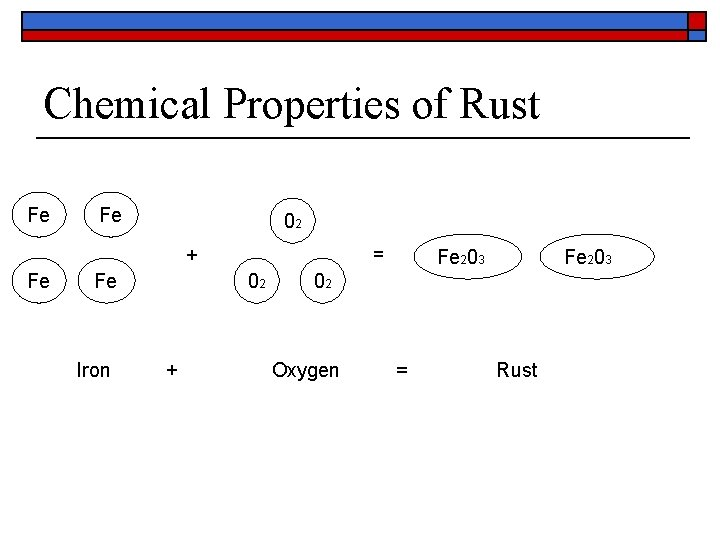Chemical Properties of Rust Fe Fe 02 + Fe Fe Iron = 02 + Fe 203 02 Oxygen = RustCorrosion o o o Not all metal can be corroded by air. The Statue of Liberty has reacted with the rainwater and air over time to go from a shiny copper color to a dull green color. It is made of copper, which will corrode in air. * A chemical property of copper is that is reacts with air to form green copper sulfate.Corrosion o Gold is a metal that will not corrode in air. Some metals tarnish, or turn black when exposed to air. Corroding and tarnishing are chemical properties related to interactions with air.* Properties o o o Gold (Au) Iron (Fe) The property of gold makes it different from iron it is because it does not rust.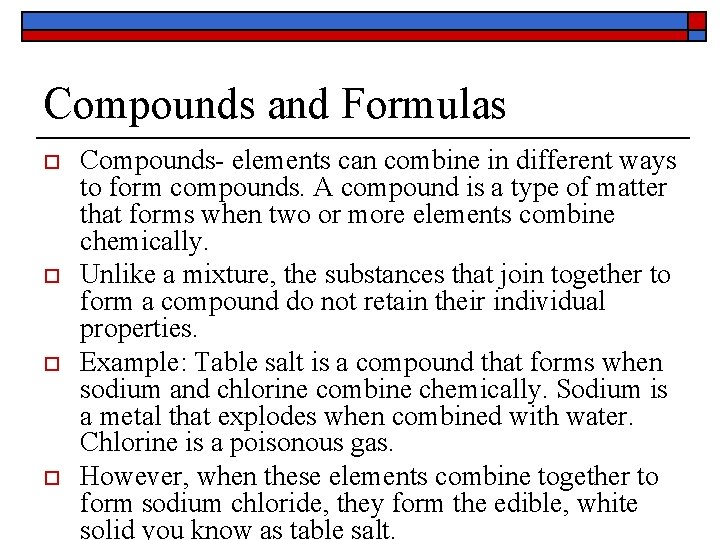Compounds and Formulas o o Compounds- elements can combine in different ways to form compounds. A compound is a type of matter that forms when two or more elements combine chemically. Unlike a mixture, the substances that join together to form a compound do not retain their individual properties. Example: Table salt is a compound that forms when sodium and chlorine combine chemically. Sodium is a metal that explodes when combined with water. Chlorine is a poisonous gas. However, when these elements combine together to form sodium chloride, they form the edible, white solid you know as table salt.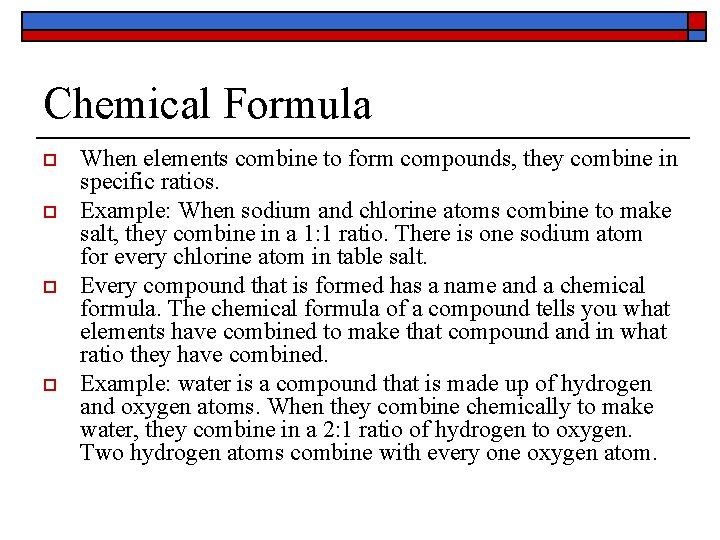Chemical Formula o o When elements combine to form compounds, they combine in specific ratios. Example: When sodium and chlorine atoms combine to make salt, they combine in a 1: 1 ratio. There is one sodium atom for every chlorine atom in table salt. Every compound that is formed has a name and a chemical formula. The chemical formula of a compound tells you what elements have combined to make that compound and in what ratio they have combined. Example: water is a compound that is made up of hydrogen and oxygen atoms. When they combine chemically to make water, they combine in a 2: 1 ratio of hydrogen to oxygen. Two hydrogen atoms combine with every one oxygen atom.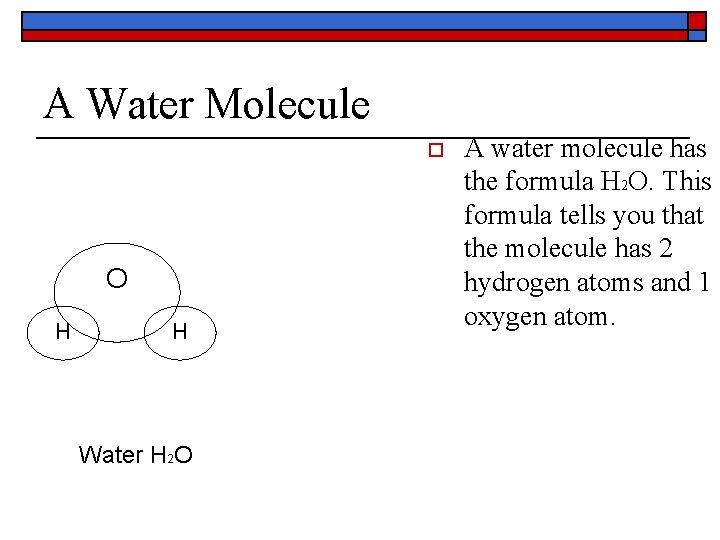A Water Molecule o O H H Water H 2 O A water molecule has the formula H 2 O. This formula tells you that the molecule has 2 hydrogen atoms and 1 oxygen atom.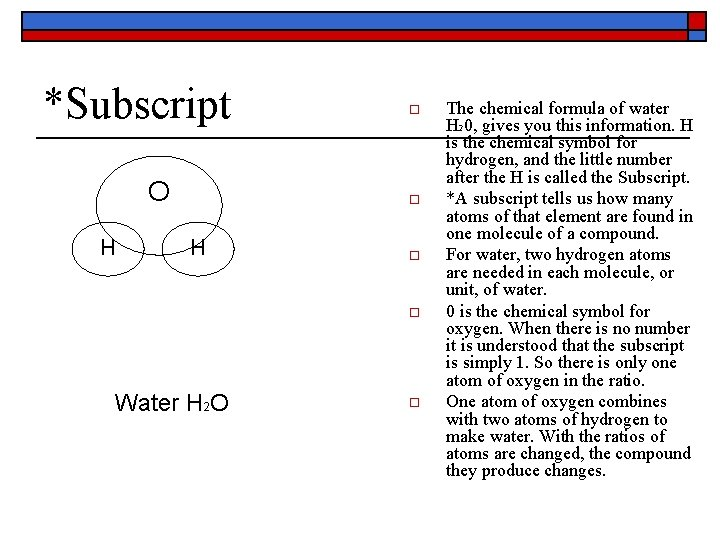*Subscript O H o o Water H 2 O o The chemical formula of water H 20, gives you this information. H is the chemical symbol for hydrogen, and the little number after the H is called the Subscript. *A subscript tells us how many atoms of that element are found in one molecule of a compound. For water, two hydrogen atoms are needed in each molecule, or unit, of water. 0 is the chemical symbol for oxygen. When there is no number it is understood that the subscript is simply 1. So there is only one atom of oxygen in the ratio. One atom of oxygen combines with two atoms of hydrogen to make water. With the ratios of atoms are changed, the compound they produce changes.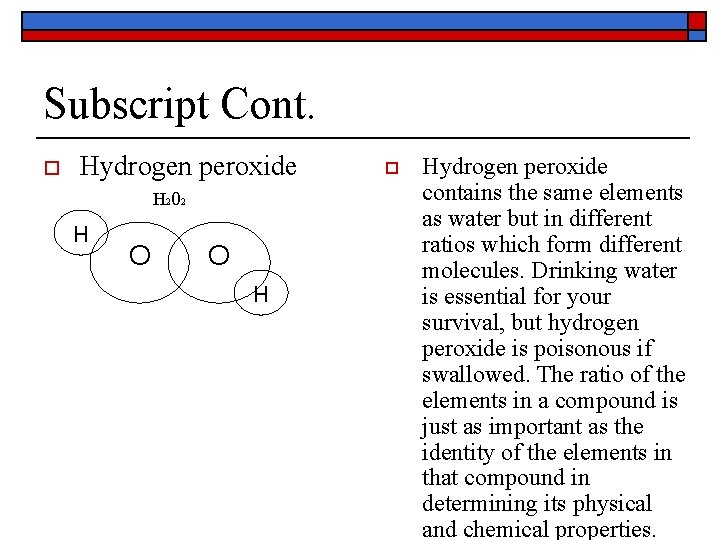Subscript Cont. o Hydrogen peroxide H 202 H O O H o Hydrogen peroxide contains the same elements as water but in different ratios which form different molecules. Drinking water is essential for your survival, but hydrogen peroxide is poisonous if swallowed. The ratio of the elements in a compound is just as important as the identity of the elements in that compound in determining its physical and chemical properties.Chemical Formulas o o o Carbon dioxide is a gas found in the air. Its chemical formula is C 02. How many different elements are in this formula? The answer is two carbon and oxygen. How many atoms of each element does it have? To answer his question, we look at the subscripts. Remember if there is no number after the element, it is understood to be 1. So there is 2 subscripts those are two oxygen atoms. How many total atoms are in one molecule of CO 2? There a total of three atoms: one carbon atom and two oxygen atoms.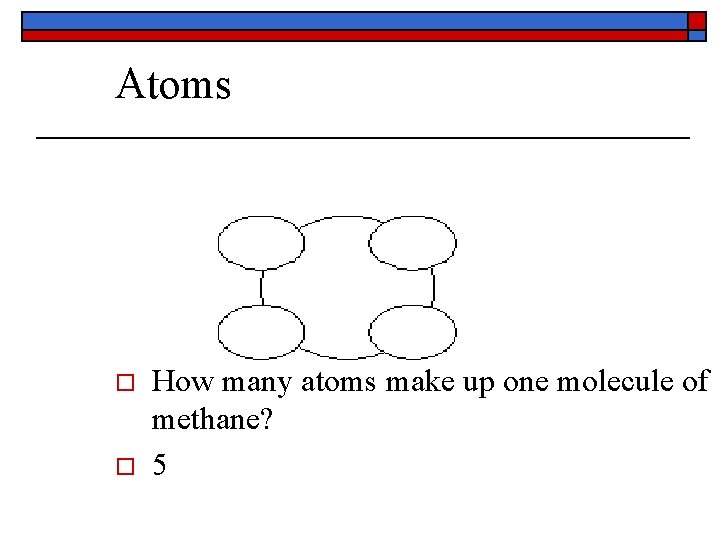Atoms o o How many atoms make up one molecule of methane? 5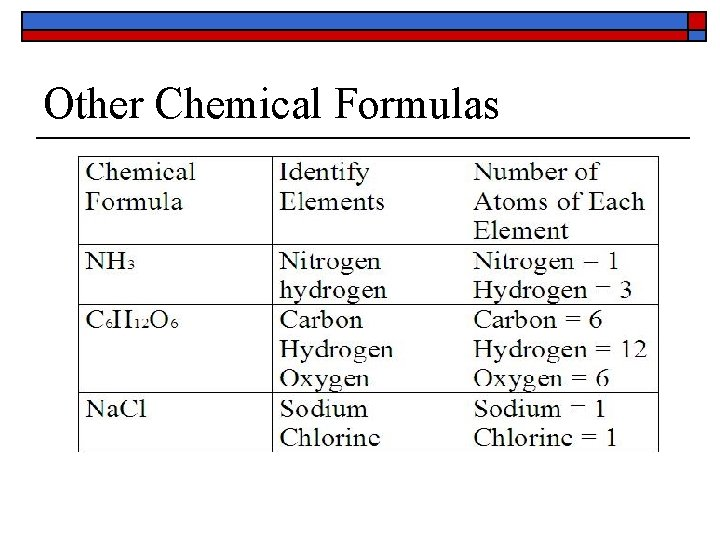Other Chemical FormulasA. Balancing Steps 1. Write the unbalanced equation. 2. Count atoms on each side. 3. Add coefficients to make #s equal. Coefficient subscript = # of atoms 4. Reduce coefficients to lowest possible ratio, if necessary. 5. Double check atom balance!!!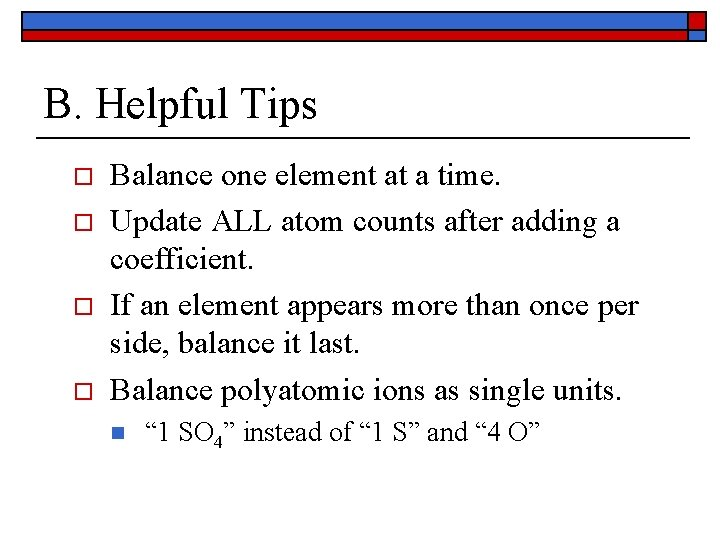B. Helpful Tips o o Balance one element at a time. Update ALL atom counts after adding a coefficient. If an element appears more than once per side, balance it last. Balance polyatomic ions as single units. n “ 1 SO 4” instead of “ 1 S” and “ 4 O”Why is there a 2 after the oxygen? _________ Oxygen is diatomic What are all the diatomic elements? Br, I, N, Cl, H, O, F _________ 4 1 12 2 10 4 2 8Yields Reactants Subscript Products Coefficient*Examples o o *The chemical formula for sodium nitrate is Na. NO 3. It is used in explosives and also as a preservative in some foods. The elements that make up sodium nitrate is: sodium, nitrogen, and oxygen. *How many total atoms are in one molecule of sodium nitrate? Na. NO 3 n n n 5 *Ammonium perchlorate is used in rocket fuels. How many atoms are in the chemical formula for ammonium perchlorate, NH 4 CIO 4? 1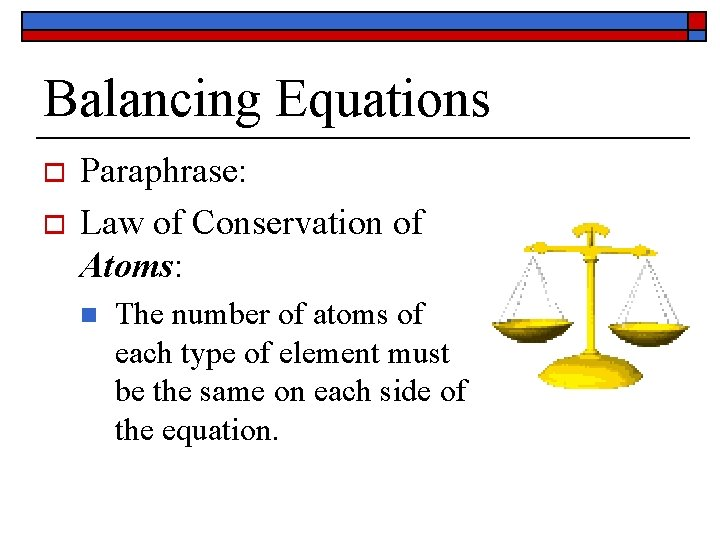Balancing Equations o o Paraphrase: Law of Conservation of Atoms: n The number of atoms of each type of element must be the same on each side of the equation.Balancing Equations n Hydrogen n H 2 o o o + oxygen + O 2 water H 2 O Hydrogen and oxygen are diatomic elements. Their subscripts cannot be changed. The subscripts on water cannot be changed.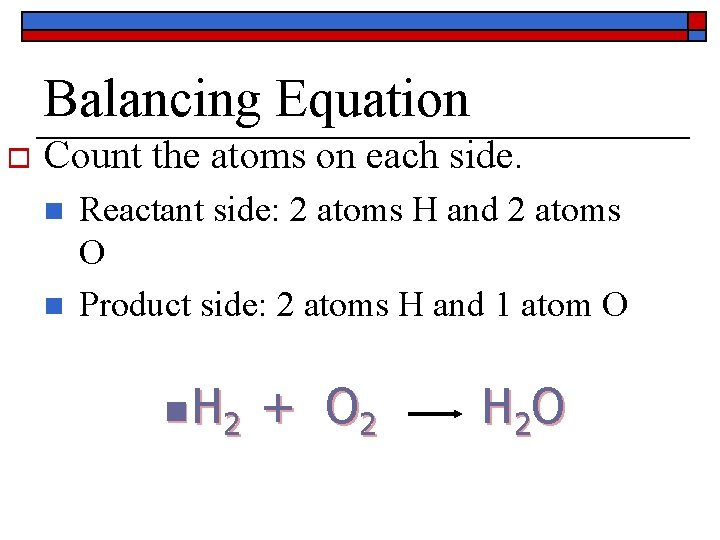Balancing Equation o Count the atoms on each side. n n Reactant side: 2 atoms H and 2 atoms O Product side: 2 atoms H and 1 atom O n H 2 + O 2 H 2 O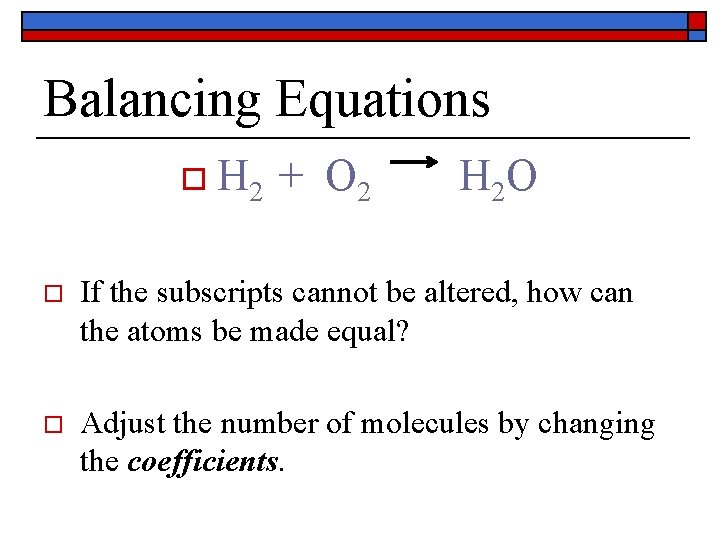Balancing Equations o H 2 + O 2 H 2 O o If the subscripts cannot be altered, how can the atoms be made equal? o Adjust the number of molecules by changing the coefficients.Balancing Equations o o o Reactants: 2 atoms of H and 2 atoms of O Products: 4 atoms of H and 2 atoms of O H is no longer balanced! n H 2 + O 2 2 H 2 O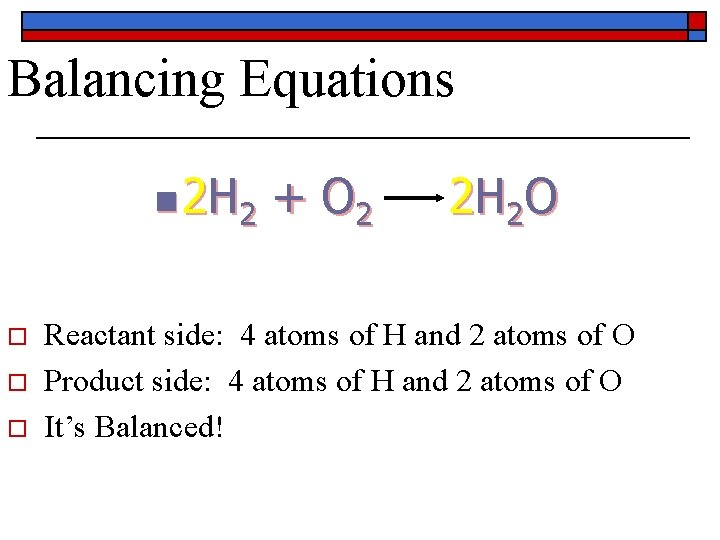Balancing Equations n 2 H 2 o o o + O 2 2 H 2 O Reactant side: 4 atoms of H and 2 atoms of O Product side: 4 atoms of H and 2 atoms of O It’s Balanced!Balancing Equations n N 2 n o + H 2 Nitrogen + hydrogen NH 3 ammonia Count atoms. n n Reactants: 2 atoms N and 2 atoms H Products: 1 atom N and 3 atoms of NH 3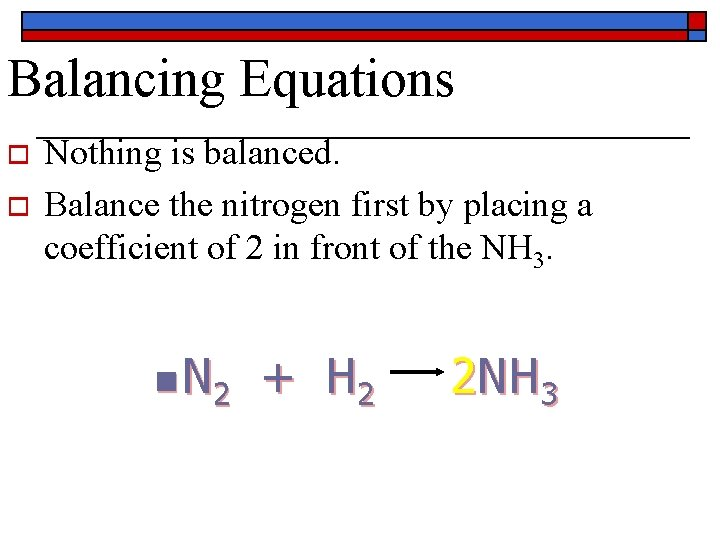Balancing Equations o o Nothing is balanced. Balance the nitrogen first by placing a coefficient of 2 in front of the NH 3. n N 2 + H 2 2 NH 3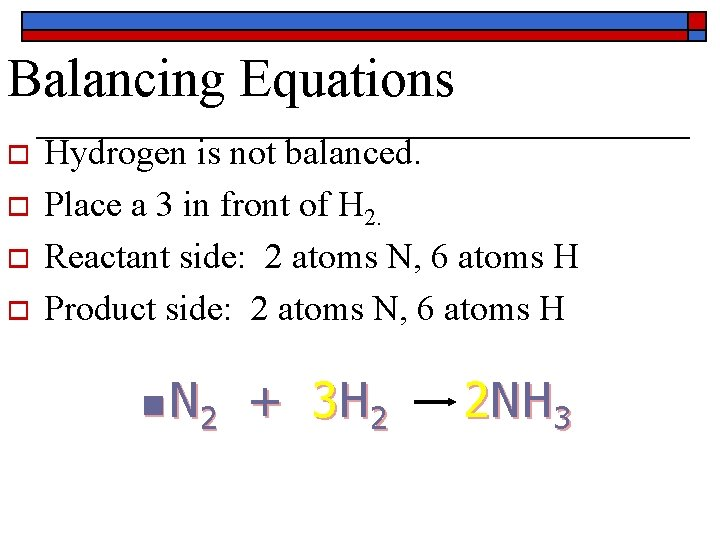Balancing Equations o o Hydrogen is not balanced. Place a 3 in front of H 2. Reactant side: 2 atoms N, 6 atoms H Product side: 2 atoms N, 6 atoms H n N 2 + 3 H 2 2 NH 3Balancing Equations o Ca 3(PO 4)2 + H 2 SO 4 + H 3 PO 4 Ca. SO 4 o Count atoms in the product. o Ca atoms – 1, S atom – 1, O atoms – 4; H atoms – 3, P atom – 1, O atoms - 4Balancing Equations o A coefficient of 2 placed in front of H 3 PO 4 which balances both hydrogen and phosphate. o Ca 3(PO 4)2 + 3 H 2 SO 4 3 Ca. SO 4 + 2 H 3 PO 4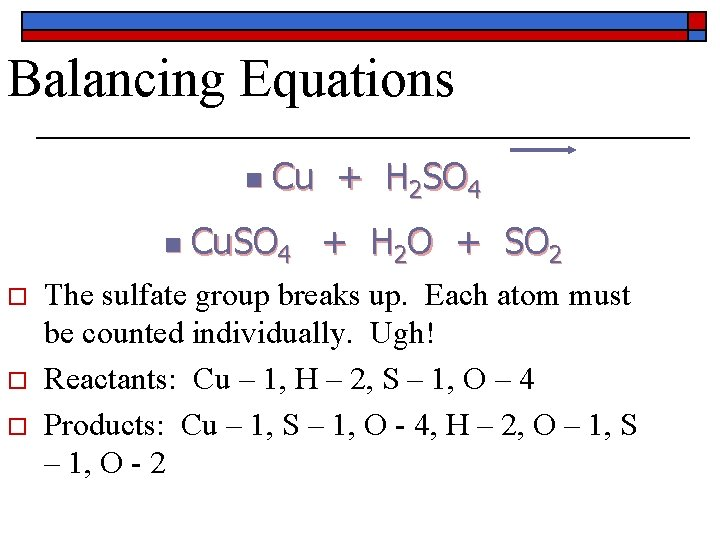Balancing Equations n n o o o Cu + H 2 SO 4 Cu. SO 4 + H 2 O + SO 2 The sulfate group breaks up. Each atom must be counted individually. Ugh! Reactants: Cu – 1, H – 2, S – 1, O – 4 Products: Cu – 1, S – 1, O - 4, H – 2, O – 1, S – 1, O - 2Balancing Equations o o o Sulfur is not balanced. Place a two in front of sulfuric acid. Count atoms: 2 H 2 SO 4 H – 4, S – 2, O 8 Cu + 2 H 2 SO 4 Cu. SO 4 + H 2 O + SO 2 o o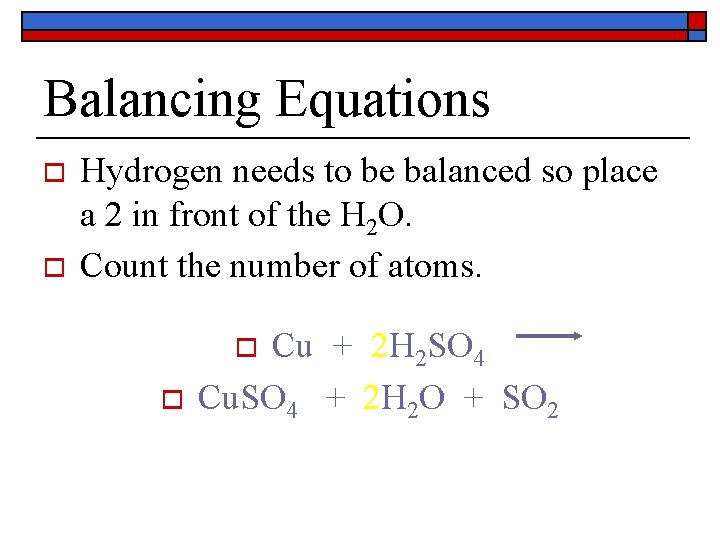Balancing Equations o o Hydrogen needs to be balanced so place a 2 in front of the H 2 O. Count the number of atoms. Cu + 2 H 2 SO 4 Cu. SO 4 + 2 H 2 O + SO 2 o oBalancing Equations o o Reactants: Cu – 1, H – 4, S – 2, O – 8 Products: Cu – 1, S – 1, O – 4, H – 4, O – 2, S – 1, O – 2 = Cu – 1, S – 2, H – 4, O – 8 o It’s balanced! Cu + 2 H 2 SO 4 Cu. SO 4 + 2 H 2 O + SO 2 o o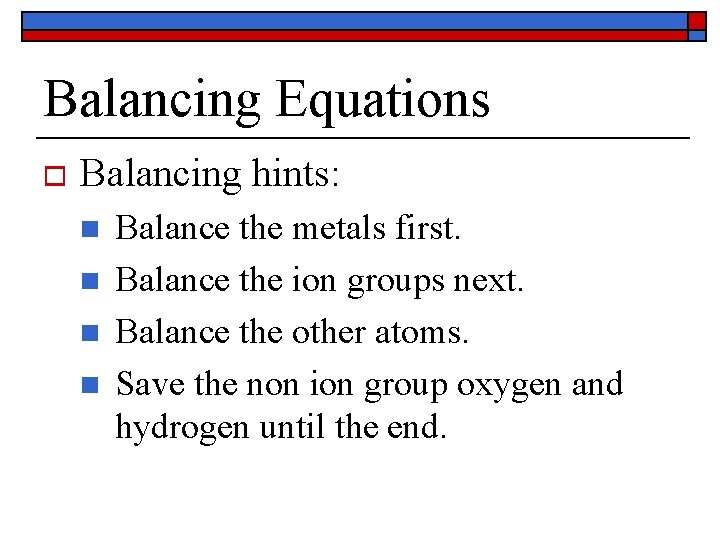Balancing Equations o Balancing hints: n n Balance the metals first. Balance the ion groups next. Balance the other atoms. Save the non ion group oxygen and hydrogen until the end.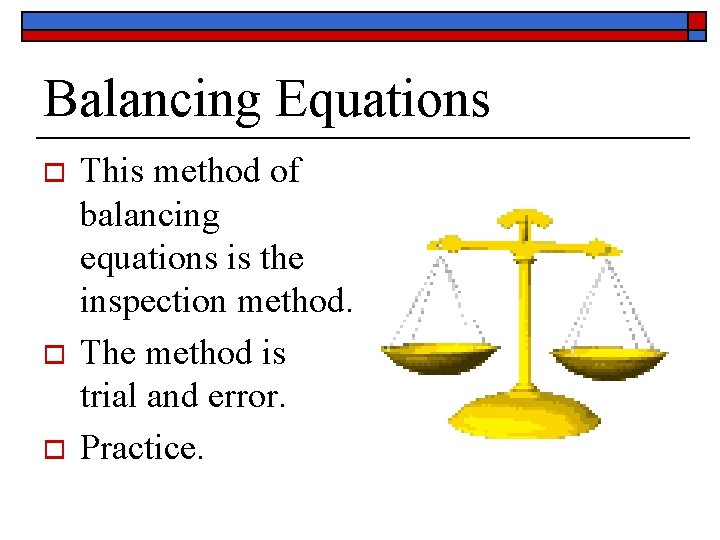Balancing Equations o o o This method of balancing equations is the inspection method. The method is trial and error. Practice.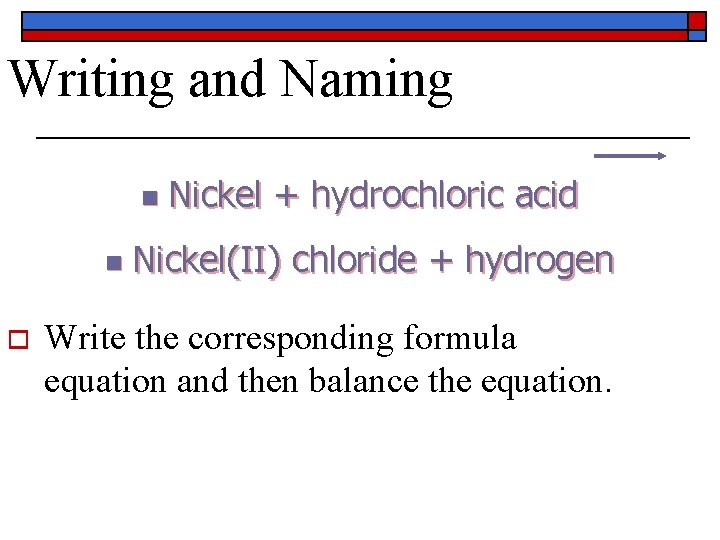Writing and Naming n n o Nickel + hydrochloric acid Nickel(II) chloride + hydrogen Write the corresponding formula equation and then balance the equation.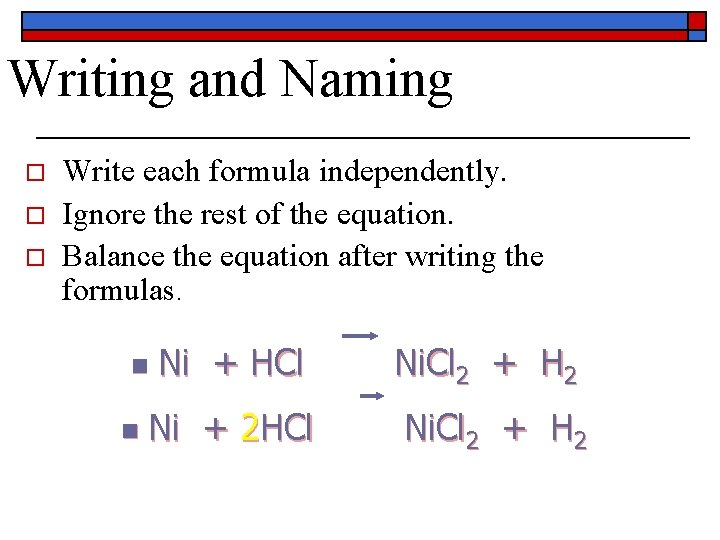Writing and Naming o o o Write each formula independently. Ignore the rest of the equation. Balance the equation after writing the formulas. Ni + HCl Ni. Cl 2 + H 2 Ni + 2 HCl Ni. Cl 2 + H 2 n n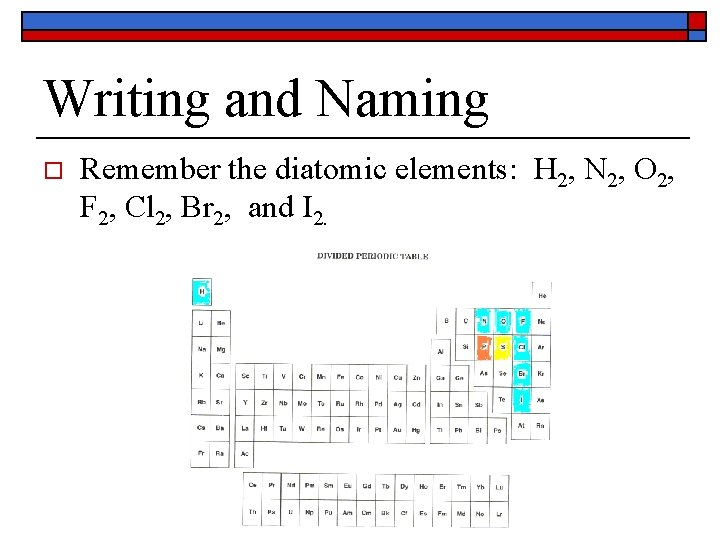Writing and Naming o Remember the diatomic elements: H 2, N 2, O 2, F 2, Cl 2, Br 2, and I 2.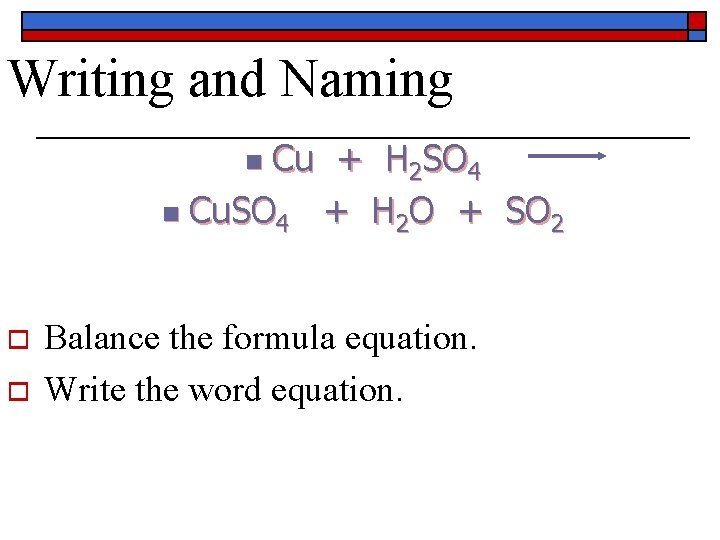Writing and Naming Cu + H 2 SO 4 n Cu. SO 4 + H 2 O + SO 2 n o o Balance the formula equation. Write the word equation.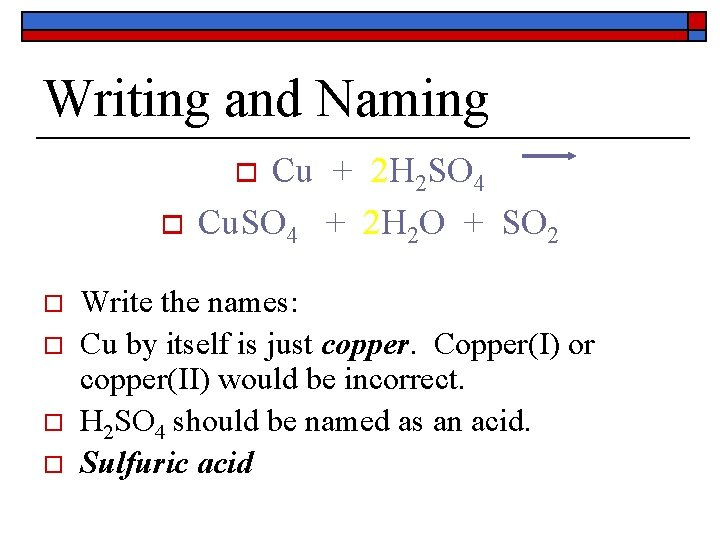Writing and Naming Cu + 2 H 2 SO 4 Cu. SO 4 + 2 H 2 O + SO 2 o o o Write the names: Cu by itself is just copper. Copper(I) or copper(II) would be incorrect. H 2 SO 4 should be named as an acid. Sulfuric acid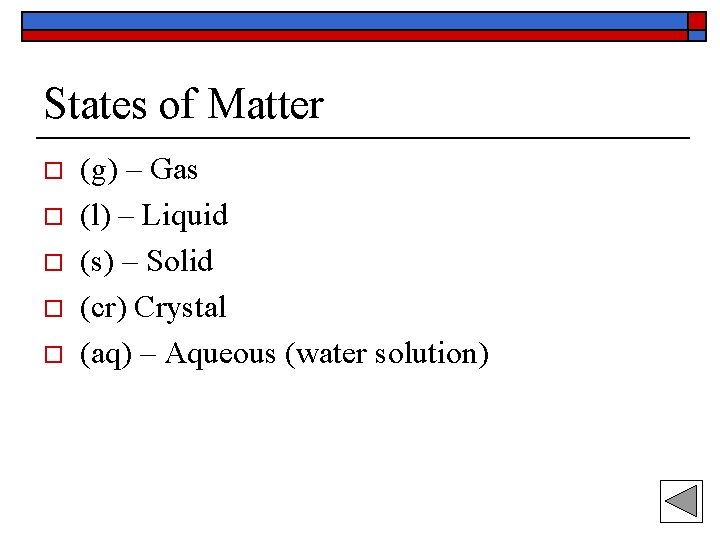States of Matter o o o (g) – Gas (l) – Liquid (s) – Solid (cr) Crystal (aq) – Aqueous (water solution)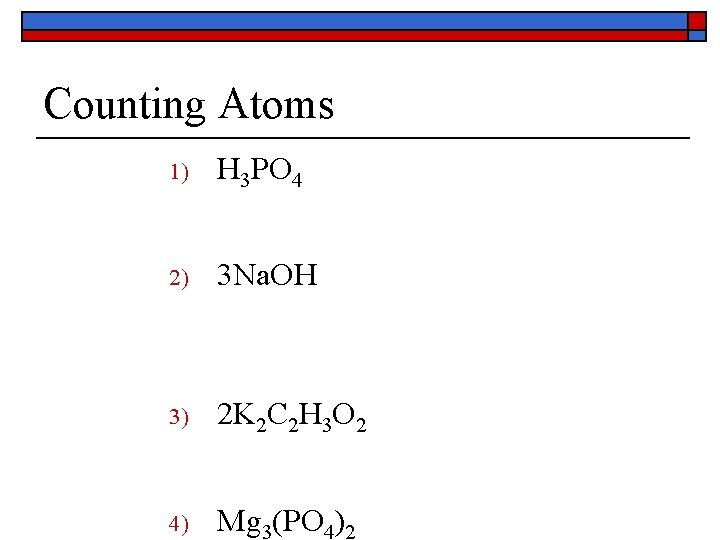Counting Atoms 1) H 3 PO 4 2) 3 Na. OH 3) 2 K 2 C 2 H 3 O 2 4) Mg 3(PO 4)2More Counting Atoms 1) 3 Al(NO 3)3 2) 3 Al 2(SO 4)3 3) 2 Ca(OH)2 4) 3 NH 4 NO 3Balanced or not? o H 2 + O 2 o CH 4 + O 2 CO 2 + H 2 O o Mg + O 2 H 2 O Mg. OCellular respiration can be described by the following chemical equation: o o o C 6 H 12 O 6 + 6 O 2 glucose oxygen 6 CO 2 + 6 H 20 + ENERGY carbon dioxide water (ATP) Respiration Clip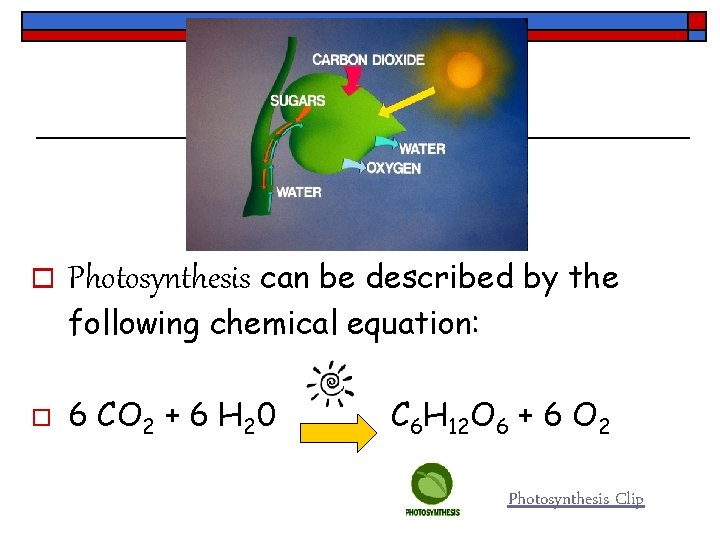o Photosynthesis can be described by the following chemical equation: o 6 CO 2 + 6 H 20 C 6 H 12 O 6 + 6 O 2 Photosynthesis ClipBalancing Equations o Practice problems on board